SUPPLEMENTAL DOCUMENT SD-4

Measurement Uncertainty for Purity Determinations in Seized Drug Analysis

FOR PART IVC – Quality Assurance/Uncertainty

A      Example 1: Control Chart Method

Introduction

The following examples demonstrate different approaches for deriving an estimate for the uncertainty associated with purity determinations.  In purity determination, uncertainty arises from two factors: sampling (including sample homogeneity) and the analysis.  Each factor is considered in the following examples.

These examples are meant to be illustrative, not exclusive.  Laboratories should develop defensible procedures that fit their operational environment and jurisdictional requirements.

In these examples, purity values are expressed as a percent composition by weight.  This can create confusion in calculations where an uncertainty contribution is provided as a relative percent.  Notes and example calculations are provided to clarify these applications.

Definitions for the terms used can be found in the SWGDRUG glossary Part IV C, Annex A or references listed below. The references also contain additional examples and detailed information regarding estimation of uncertainty.

The following examples should not be directly applied to methodology used without first considering the specific purpose of a method and its relevant operational environment.  It is assumed that quantitative methods have been validated as per SWGDRUG recommendations.

# A         Example 1: Control Chart Method

Scenario:  The laboratory is required to determine the percent purity of a single bag of solid material, weighing approximately 3 grams and previously determined to contain cocaine. In this example, measurement uncertainty is calculated using control chart data obtained from a measurement assurance process that mimics casework samples as closely as possible.  Sample homogenization is tested to ensure the laboratory’s homogeneity criteria are met.

## A.1      Sampling Uncertainty Considerations

After all of the solid material has been homogenized, duplicate samples are collected and analyzed.  The laboratory has determined that the purity difference between these two results must be equal to or less than the control limits (± 3 standard deviations) from the control chart. This process is used to establish the homogeneity of the sample.

## A.2      Methodology

The control chart derived from the analysis of a measurement assurance sample is used to capture contributions from analyst, matrix, environment, and other factors.  The use of the measurement assurance sample control chart will inevitably result in some contributions being counted twice but these are difficult to isolate.  This is a conservative approach but the likely overestimation of uncertainty was deemed acceptable by the laboratory.

The laboratory is using a validated method that has been shown to have no significant bias.  A method uncertainty was established as part of the method validation and has a value of 0.9%relative.  For this example, the laboratory established this value by analyzing 5 different samples with known purity.  These five samples were analyzed over five days to establish figures of merit such as repeatability.

The laboratory uses a solid commercial reference material for preparation of calibration solutions. The analysis report states the purity to be ≥ 99.0% and the reference material is stored as per manufacturer’s recommendation.

Long term variability associated with method performance is captured by the control chart.  The standard deviation obtained from the control chart is calculated from the 100 most recent points, which in this example is 2.1%relative. Including both the control chart and method validation contributors will result in some overlap, which is again accepted as a conservative approach.

Verification of homogeneity:

Sample #1 purity: 27.8%purity

Sample #2 purity:  28.5%purity

Average: 28.2%purity

|27.8%purity - 28.5%purity|/28.2% *100 = 2.5%relative

This value is smaller than the control limits (±3SD or 3 x 2.1 = 6.3%relative) established by the control chart, demonstrating acceptable homogeneity.

These results are interpreted to mean that the variability seen between the two duplicate samples is less that the variability of the validated method as demonstrated by the control chart.  The sample is then acceptably homogeneous and the duplicate results can be averaged to obtain a reported value.

If the difference between these replicate values falls outside the range established by the control chart, the bulk sample is considered to be inhomogeneous.  In this instance, the bulk sample must be further homogenized or the uncertainty budget would have to be adjusted to account for the inhomogeneity.

## A.3      Uncertainty Budget Table

 Factors Valuea Distribution Standard uncertainty (u), relative percent Index (Relative % contribution)b Calibrator uncertainty 0.5% Rectangularc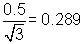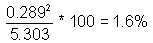Control chart 2.1% Normal 2.1 83.2 Method uncertaintyb 0.9% Normal 0.9 15.3 Subtotal of individual u values: (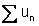): 3.289 Sum of relative contributions: 100% Subtotal of squared u values: (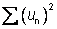): 5.303

a. Relative percentage values

b. From method validation (repeatability)

c. A rectangular distribution was selected for calibrator uncertainty because the purity of the reference material is stated such that the range of possible concentrations is 99.0-100%.  The extremes of the rectangular distribution are 99.0 and 100.  The value of a (half of the range of a rectangular distribution) is 1.0/2 = 0.5%.

## A.4      Calculation of combined standard uncertainty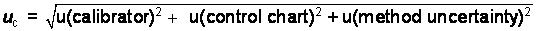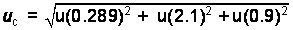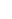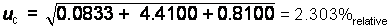## A.5      Calculation of expanded uncertainty

The expanded uncertainty is expressed mathematically as:

U = k*uc

In this case the uc is calculated as a relative percentage and must be converted to an absolute percent of the experimentally determined mean value:

2.303/100 = 0.02303 * experimentally determined value = 0.02303 * 28.2%purity = 0.6494%purity

Using a coverage factor k = 2 (confidence level of approximately 95%, assuming the %purity follows a normal distribution):

U = 2 * 0.6494%purity = 1.30%purity

Using a coverage factor k = 3 (confidence level of approximately 99% assuming the %purity follows a normal distribution):

U = 3 * 0.6494%purity = 1.95%purity

## A.6      Results

A.6.1   28.2%purity ± 1.3%purity (k=2)

A.6.2   28.2%purity ± 2.0%purity (k=3)

# B         Example 2: Use of Proficiency Test Data to Estimate Uncertainty in Combination with Control Chart Data.

Scenario:  The scenario is the same as in Example A, except this example also includes contributions for uncertainty associated with reference material and matrix effects using historical proficiency test data collected over several years across a multi-laboratory system. This is a conservative approach but the likely overestimation of uncertainty is deemed acceptable by the laboratory.

The proficiency test is performed annually across multiple laboratories which use different analytical techniques and different batches of reference materials for quantitation. The controlled substance in the reference material is cocaine. The proficiency data set represents 6 test events over the past six years with 22 participating laboratories. In this example, the consensus value is used as the accepted value for the %purity of the proficiency test sample.

Data for this example is shown in the following table.  All purities are given in units of %purity.

 All participating laboratories Laboratory #1 Year1 Consensus value (Cref)2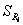(%)3 Proficiency test result Bias (% error relative to the assigned value)4 2012 17.9 4.8 18.7 +4.5 2011 29.3 2.6 28.8 -1.7 2010 23.3 7.9 24.2 +3.9 2009 26.1 5.1 26.0 -0.4 2008 13.7 9.5 13.3 -2.9 2007 33.5 3.3 34.1 +1.8 Mean: 5.55

1. For each year, m = 22 participants

2. Consensus value (mean) of %purity obtained from proficiency test event

3. Reproducibility standard deviation expressed as relative percentage values.  This is the standard deviation for the %purity values obtained within a given year for m = 22

4. Sample calculation for 2012: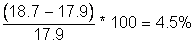5. Arithmetic mean reproducibility standard deviation (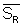) calculated over i years.

## B.1      Sampling Uncertainty Considerations:

The scenario is the same as in Example A.

## B.2      Methodology

The scenario is the same as in Example A.  The calibrator contribution is retained because Laboratory #1 is using a different reference material for calibration for this case sample as was used for the proficiency tests and for the control chart.

This example includes two additional uncertainty contributions:

1) Method bias for Laboratory #1: A contribution derived from Laboratory #1’s performance on the proficiency tests as compared to the consensus values.

2) Standard uncertainty of the mean () value: A contribution from the uncertainty associated with the consensus values of the proficiency tests.

Information regarding the determination of these two contributions and how they are evaluated may be found in the references below

## B.3      Uncertainty Budget Table

 Factors Valuea Distribution Standard Uncertainty (u), Relative Percent Index (Relative % Contribution) Calibrator uncertainty for calibrator used in Laboratory #1 0.5% Rectangularb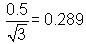0.6 Control chart maintained by Laboratory #1 2.1% Normal 2.1 30.7 Method bias for Laboratory #1c 2.9% not applicabled 2.9 58.6 Standard uncertainty of the mean (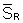) valuee 1.2% Normal 1.2 10.0 Subtotal of individual u values: (): 6.489 Sum of relative contributions: 100% Subtotal of squared u values: (): 14.34

a. Relative percentage values.

b. The width of the rectangular distribution is 1.0/2 = a = 0.5; another way to express the purity of the calibratior is 99.5 +/- 0.5%

c. Expressed as root mean square (RMS):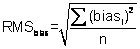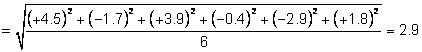; n represents the number of proficiency tests

d. The RMS is a measure which is independent from a statistical distribution.

e. Calculated as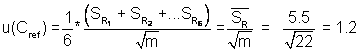;represents the mean reproducibility standard deviation of six proficiency tests; m is the number of proficiency test participants

## B.4      Calculation of combined standard uncertainty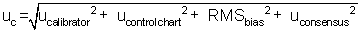In this example, the contribution from the calibrator could be excluded because of its minimum contribution to the total combined uncertainty.  The decision to remove a contribution should be made after all uncertainty contributions are evaluated.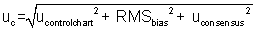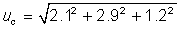uc = 3.8%relative

## B.5      Calculation of expanded uncertainty

Expanded uncertainty is expressed mathematically as:

U = k*uc

In this case, uc is calculated as a relative percentage and must be converted to an absolute percent of the experimentally determined mean value:

3.8/100 = 0.038 * experimentally determined value =

0.038 * 28.2%purity = 1.1%purity

Using a coverage factor k = 2 (confidence level of approximately 95% and assuming the %purity follows a normal distribution):

U =2*1.1%purity = 2.2%purity

Using a coverage factor k = 3 (confidence level of approximately 99% assuming the %purity follows a normal distribution):

U = 3*1.1%purity = 3.3%purity

## B.6      Results

B.6.1   28.2%purity ± 2.2%purity (k=2)

B.6.2   28.2%purity ± 3.3%purity (k=3)

# C         Example 3: Single Case Quantitation Utilizing Replicate Samplings

Scenario:  The laboratory is required to determine the percent purity of a single item of solid material, weighing 3 grams and previously determined to contain heroin.  The laboratory has a documented sampling plan and although it does not routinely quantitate heroin, the laboratory has a method available for general drug quantitation using a multipoint calibration curve.  Homogeneity of the bulk case sample is not assumed and variations in composition will be reflected in the standard deviation of the replicates that is included in the combined uncertainty.

The laboratory elects not to use a control chart.  Two samples are taken from a QC material with a known 79.3%purity.  These two samples are prepared such that the concentration of one is near the lower limit of the working range and the concentration of the other is near the upper limit of the working range.  The target analyte concentration in the case samples must fall within the range bracketed by these two QC samples.  All samples (calibration, QC and case) are analyzed contemporaneously. The difference in the experimentally determined purities of both QC solutions must meet pre-defined laboratory criteria.

In this example the laboratory has elected to analyze 6 samples of the case material.

The laboratory has a general acceptance criterion for accuracy of ± 5.0%relative for purposes such as method validation.  In this example, the laboratory has set the acceptance criteria for the QC solutions to be ± 5.0%relative difference between the known value and the experimentally determined value.

## C.1      Sampling Uncertainty Considerations

Six samples are taken from case materials to account for variations in the composition of the bulk.

## C.2      Methodology

Uncertainty is estimated by considering contributions from two sources:  1) The accuracy of the quantitative procedure as verified by the results for the two QC solutions; and 2) the variability of the six samples.

It is assumed that if the measured purity for the QC solutions both fall within ± 5.0%relative of the accepted true value, the maximum contribution to uncertainty arising from the method accuracy is ± 5.0%relative.  In this example, acceptable accuracy was demonstrated as follows:

## C.3      Conditions

Calibration curve range: 0.100 mg/ml – 1.500 mg/ml

QC sample accepted true value (%purity):  79.3

5%relative of 79.3% = 4.0%

Acceptable range of QC Sample results:  75.3 - 83.3%

 Amount Weighed / Volume Experimentally Determined Concentration Calculated Percent Purity of QC Sample QC Solution 1 26.0 mg / 100 ml 0.214 mg/ml 82.3% QC Solution 2 186.7 mg / 100 ml 1.423 mg/ml 76.2%

Experimentally determined %purity Solution 1:  82.3% ACCEPTED

Experimentally determined %purity Solution 2:  76.2% ACCEPTED

Working range determined by QC solutions: 0.214 mg/ml – 1.423 mg/ml

Because both calculated %purity values fall within ± 5.0%relative, a conservative approach is taken to set method accuracy at 5.0%.

Had either or both of these values fallen outside of the ± 5.0%relative range, the laboratory would have to reassess the approach and procedure.

## C.4      Calculation of combined standard uncertainty

Results for sample replicates:

 % purity Sample 1 26.0 Sample 2 24.9 Sample 3 25.0 Sample 4 27.0 Sample 5 25.4 Sample 6 27.0 Mean 25.88 s 0.947 Relative standard deviation 3.66%

In this example, the laboratory elects to consider the tolerance value of ±5.0%relative as a rectangular distribution in which a = 5.0 and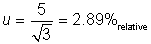This is taken to represent the contribution from the method bias.  Including this contribution is a conservative approach which will likely result in an overestimation of the uncertainty.

It should be noted that other ways exist of calculating and expressing the systematic component of error for this analysis.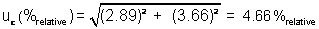## C.5      Calculation of expanded uncertainty

In this case, the expanded uncertainty is calculated using the Student’s t value rather than k because the number of samples analyzed is relatively small.

In this example, the contribution from the systematic component, 2.89, is treated as a constant.  As a result, the value of the Student’s t is determined based only on the degrees of freedom associated with the random component determined by analysis of the 6 replicates, or at 5 degrees of freedom.

This represents a conservative approach. The Student’s t value is selected at the 95% and 99% confidence levels.

In this case, uc is calculated as a relative percentage and must be converted to an absolute percent of the experimentally determined mean value:

4.7/100 = 0.047 * experimentally determined value =

0.047 * 25.9%purity = 1.2%purity

95% confidence level

U = t*uc

U = 2.571 * 1.2%purity =  3.1%purity

99% confidence level:

U = 4.032 * 1.2%relative =  4.8%relative

Alternatively, a laboratory may elect to calculate the effective degrees of freedom to determine the value of the Student’s t, which will fall between 2 and 2.571.  Selection of the value of 2.571 based on 5 degrees of freedom in this case will likely result in an overestimation of the uncertainty.

## C.6      Results

C.6.1   25.9% ± 3.1% (95% confidence level)

C.6.2   25.9% ± 4.8% (99% confidence level)

## D.1      Books

D.1.1 Dieck, R.H. 2007. Measurement Uncertainty: Methods and Applications. 4th ed. Research Triangle Park, NC: The Instruments, Systems, and Automation Society (ISA).

D.1.2  Kirkup, L., and R. B. Frenkel. 2006. An Introduction to Uncertainty in Measurement. Cambridge, UK: Cambridge University Press.

D.1.3  Taylor, J.R. 1997. An Introduction to Error Analysis: The Study of Uncertainties in Physical Measurements. Sausalito, CA: University Science Books.

## D.2      Journal articles and reviews

D.2.1 ASTM, Standard Practice for Estimating and Monitoring the Uncertainty of Test Results of a Test Method in a Single Laboratory Using a Control Sample Program. ASTM International: West Conshocken, PA, 2007; Vol. E2554-07.

D.2.2 Baldan, A.; van der Veen, A. M. H.; Prauss, D.; Recknagel, A.; Boley, N.; Evans, S.; Woods, D., Economy of proficiency testing: reference versus consensus values. Accredit. Qual. Assur. 2001, 6 (4-5), 164-167.

D.2.3 Brown, R. J. C., and M. J. T. Milton. 2007. Developments in accurate and traceable chemical measurements. Chemical Society Reviews 36 (6):904-913.

D.2.4 Burns, M. 2004. Current practice in the assessment and control of measurement uncertainty in bio-analytical chemistry. Trac-Trends in Analytical Chemistry 23 (5):393-398.

D.2.5 Konieczka, P. 2007. The role of and the place of method validation in the quality assurance and quality control (QA/QC) system. Critical Reviews in Analytical Chemistry 37 (3):173-190.

D.2.6 Linsinger, T. P. J. 2008. Use of recovery and bias information in analytical chemistry and estimation of its uncertainty contribution. Trac-Trends in Analytical Chemistry 27 (10):916-923.

D.2.7 Meyer, V. R. 2007. Measurement uncertainty. Journal of Chromatography A 1158 (1-2):15-24.

D.2.8 Populaire, S., and E. C. Gimenez. 2006. A simplified approach to the estimation of analytical measurement uncertainty. Accreditation and Quality Assurance 10 (9):485-493.

D.2.9 Pozivil, M., W. Winiger, S. Wunderli, and V. R. Meyer. 2006. The influence of climate conditions on weighing results. Microchimica Acta 154 (1-2):55-63.

D.2.10 Rios, A., and M. Valcarcel. 1998. A view of uncertainty at the bench analytical level. Accreditation and Quality Assurance 3 (1):14-19.

D.2.11 Wong, S. K., Purity verification and measurement uncertainty. Accredit. Qual. Assur. 2010, 15 (6), 337-341.

## D.3      On-line resources

D.3.1 European Co-Operation for Accreditation, Publication EA 4/02, Expression of the Uncertainty of Measurement in Calibration, Dec 1999.

D.3.2 EURACHEM. Quantifying Uncertainty in Analytical Measurement, 2007. http://www.measurementuncertainty.org/index.html.

D.3.3 JCGM. JCGM 100-2008: Evaluation of measurement data (GUM 1995 with minor corrections). JCGM: Joint Committee for Guides in Metrology, 2008.

D.3.4 NIST 2007 Technical Note 1297, Guide for Evaluating and Expressing the Uncertainty of NIST Measurement Results National Institute of Science and Technology, U.S. Department of Commerce.

http://www.nist.gov/pml/pubs/tn1297/index.cfm

D.3.5 NIST. 2006. NIST/SEMATECH e-Handbook of Statistical Methods: National Institute of Science and Technology, U.S. Department of Commerce. http://www.itl.nist.gov/div898/handbook.

D.3.6 NIST. 2006. The NIST Reference on Constants, Units, and Uncertainty  2009. Available from http://physics.nist.gov/cuu/Uncertainty/index.html.

D.3.7 NIST 1994. NIST Technical note 1297Guidelines for Evaluating and Expressing the Uncertainty of NIST Measurement Results. National Institute of Science and Technology, U.S. Department of Commerce. http://physics.nist.gov/Pubs/guidelines/contents.html.

End of Document

 The approximate confidence levels given here assume that the quantities for which expanded uncertainties are being computed each approximately follow a normal distribution.  If this assumption does not hold, the actual confidence level attained for these uncertainty intervals may be lower or higher than the conventional 95% or 99% levels for this context.

 ASTM, Standard Guide for Reporting Uncertainty of Test Results and Use of the Term Measurement Uncertainty in ASTM Methods. ASTM International: West Conshocken, PA, 2008; Vol. E2655-08.

Magnusson, B., N. T., et al. (2003). Handbook for Calculation of Measurement Uncertainty in Environmental Laboratories, Nordtest Project.

Ramsey, M. H.; Geelhoed, B.; Wood, R.; Damant, A. P., Improved evaluation of measurement uncertainty from sampling by inclusion of between-sampler bias using sampling proficiency testing. Analyst  (2011), 136 (7), 1313-1321.

 The approximate confidence levels given in this document (SD-4) assume that the quantities for which expanded uncertainties are being computed each approximately follow a normal distribution.  If this assumption does not hold, the actual confidence level attained for these uncertainty intervals may be lower or higher than the desired levels of 95% or 99%

 Kimothi, S. K., The Uncertainty of Measurements: Physical and Chemical Metrology: Impact and Analysis. ASQ Press (American Society for Quality): Milwaukee, WI, 2002; p 391.

Natrella, M. G., Experimental Statistics, Section 23-4. U.S. Government Printing Office: Washington, D.C., 1963.

Weitzel, M. L. J., The estimation and use of measurement uncertainty for a drug substance test procedure validated according to USP < 1225 >. Accredit. Qual. Assur. 2012, 17 (2), 139-146.

 See Annex G of the GUM (JGCM 100:2008)